Question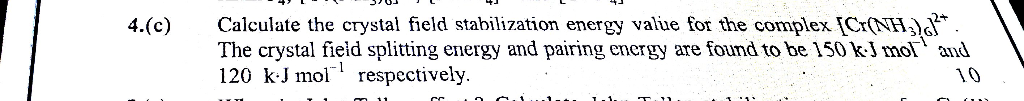Oxidation State for Chromium in the complexis (+2) and valance shell electronic configuration is.

Now d-orbital can have two electronic configuration, such as high spinor low spindepending upon the splitting power of ligands. If the ligand is weak field ligand, the complex will be high spin complex. If the ligand is strong field ligand, the complex will be low spin complex.

Here comes a factor Pairing energywhich is the energy penalty for putting two electrons in the same orbital, resulting from the electrostatic repulsion between electrons.

Forconfiguration first three electrons will go to theorbitals. If the crystal field splitting energyis lower than the pairing energy then the fourth electron will go to theorbital, hence generate a high spin complex. If the crystal field splitting energyis higher than the pairing energy then the fourth electron will go to theorbital, hence we will end up with low spin complex.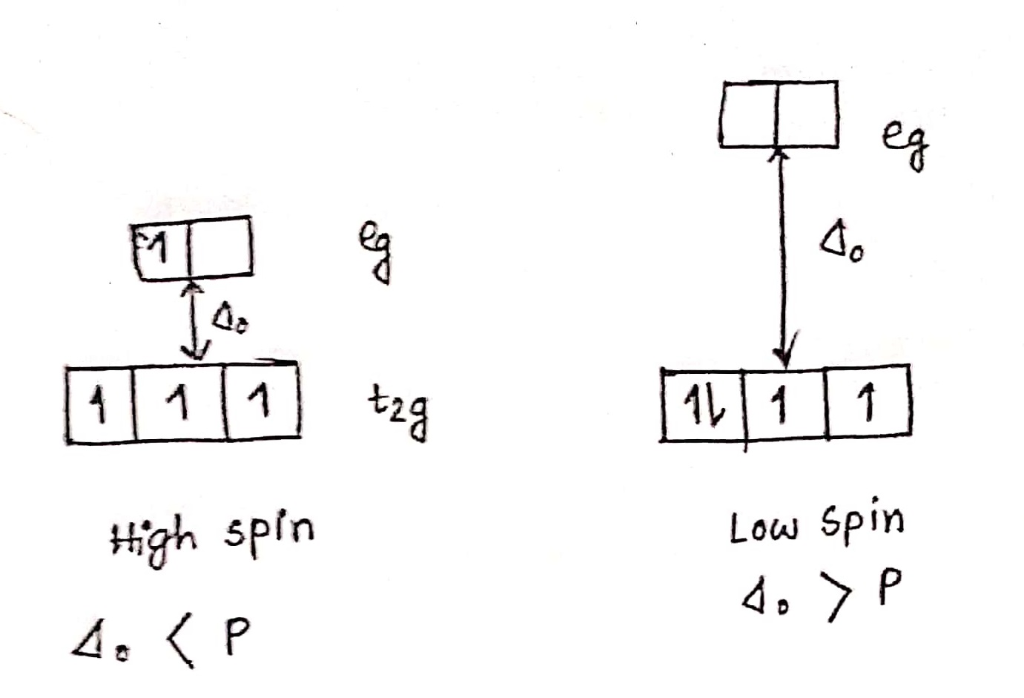In this complex,is acting as a strong field ligand as it is given in the question that the crystal field splitting energyis higher than the pairing energy, hence the complex is a low spin complex.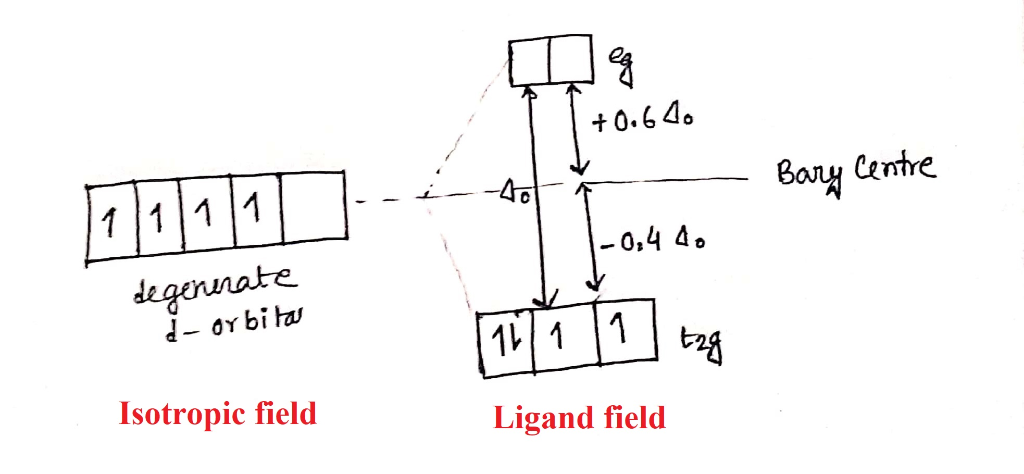Crystal Field Stabilization Energy (CFSE) =* number of t2g electrons +* number of eg electrons + P * number of pair of paired electrons =* 4 +* 0 + P * 1 ==P * number of pair of paired electrons = P * 0 = 0 kJ mol-1

CFSE ==[ANSWER]

#### Earn Coins

Coins can be redeemed for fabulous gifts.

Similar Homework Help Questions
• ### A ?1 octahedral complex is found to absorb visible light, with the absorption maximum occurring at 499 nm . Calculate the crystal-field splitting energy, Δ , in kJ/mol.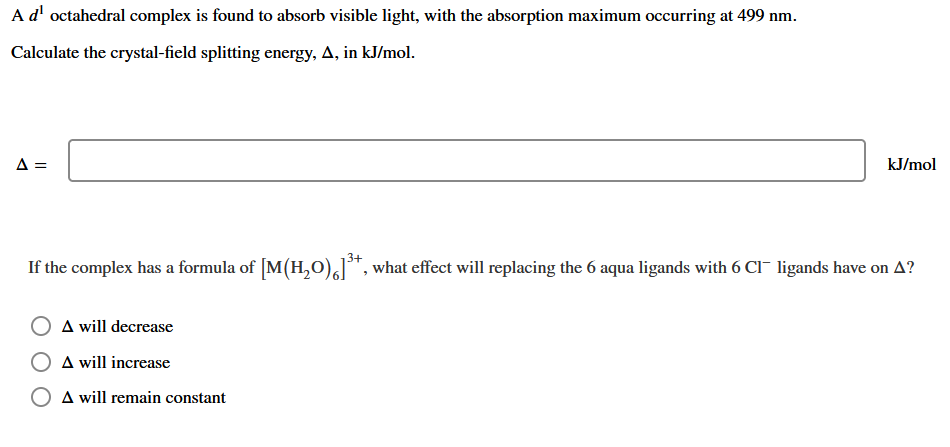A ?1 octahedral complex is found to absorb visible light, with the absorption maximum occurring at 499 nmCalculate the crystal-field splitting energy, Δin kJ/mol.

• ### Estimate the crystal field stabilization energy for the octahedral ion hexacyanocobaltate(III), if the wavelength of maximum...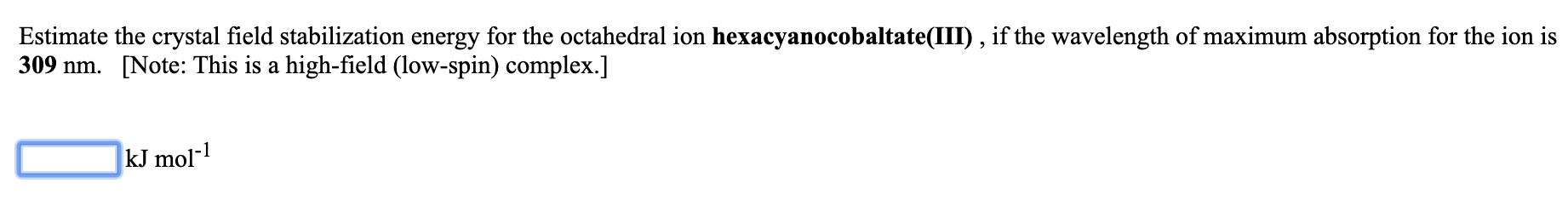Estimate the crystal field stabilization energy for the octahedral ion hexacyanocobaltate(III), if the wavelength of maximum absorption for the ion is 309 nm. [Note: This is a high-field (low-spin) complex.] kJ mol-1

• ### Estimate the crystal field stabilization energy for the octahedral ion hexafluorocobaltate(III), if the wavelength of maximum...

Estimate the crystal field stabilization energy for the octahedral ion hexafluorocobaltate(III), if the wavelength of maximum absorption for the ion is 710 nm.   [Note: This is a weak-field (high-spin) complex.] _____ kJ mol-1

• ### inorganic chem

3)      Consider [Co(H2O)6]2+   and [Cr(C2O4)3]3-   complex ionsa)       Calculate the splitting energies of these complex ions.b)      Compare your results with pairing energies of these metal ions and decide whether the ligands are weak field or strong field ligands.c)       Calculate the crystal field stabilization energy for each compound.Complexλ1λ2λ3Pairing   Energy (Kj/mol)[Co(H2O)6]2+510460-250. 0[Cr(C2O4)3]3-565415266244.3

• ### The extent of crystal field splitting is often determined from spectra. Given the wavelength (7.) of...The extent of crystal field splitting is often determined from spectra. Given the wavelength (7.) of maximum absorption, 2. = 295 nm, find the crystal field splitting energy (A), in kJ/mol, for the complex ion (Rh(NH3).13+. kJ/mol

• ### (8 pts) 13. a. Give the crystal field splitting diagram for the complex ion, [Cr(CN)! with...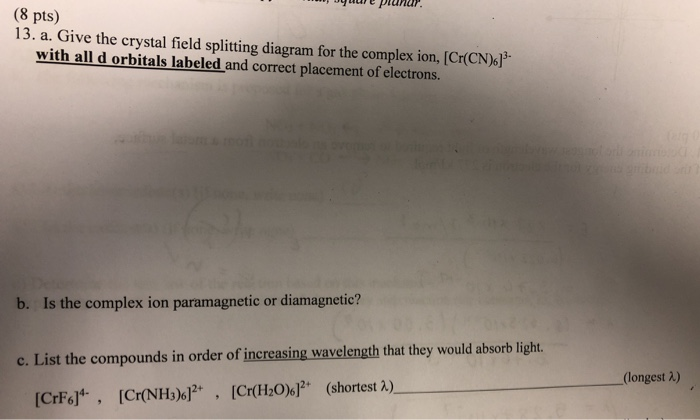(8 pts) 13. a. Give the crystal field splitting diagram for the complex ion, [Cr(CN)! with all d orbitals labeled and correct placement of electrons. b. Is the complex ion paramagnetic or diamagnetic? c. List the compounds in order of increasing wavelength that they would absorb light. (longest 2) (shortest A) [Cr(H2O)6]2. [CMNHJs产 [CrF6]4-, ,

• ### The complex [Fe(H2O)6]3+ has a maximum absorbance at 724 nm. a.Calculate the crystal field splitting energy,...

The complex [Fe(H2O)6]3+ has a maximum absorbance at 724 nm. a.Calculate the crystal field splitting energy, in kJ, for the complex. b.Predict the colour of the complex.

• ### The wavelength of light (in nanometers) that is absorbed by a complex that has a crystal-field...

The wavelength of light (in nanometers) that is absorbed by a complex that has a crystal-field splitting energy of 3.30×102 kJ/mol is _____. (h = 6.626 × 10−34 J·s, c = 3.00 × 108 m/s)

• ### What is spin pairing energy (SPE)? How is it related to crystal field splitting energy? Relate the splitting energy for...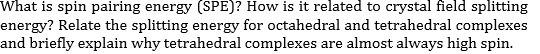What is spin pairing energy (SPE)? How is it related to crystal field splitting energy? Relate the splitting energy for octahedral and tetrahedral complexes and briefly explain why tetrahedral complexes are almost always high spin

• ### Arrange the following complex ions by increasing crystal field splitting energy. [Co(NH) [Co(CO), [Co(OH)34- [Co(CN),J4- [COCH,O),P...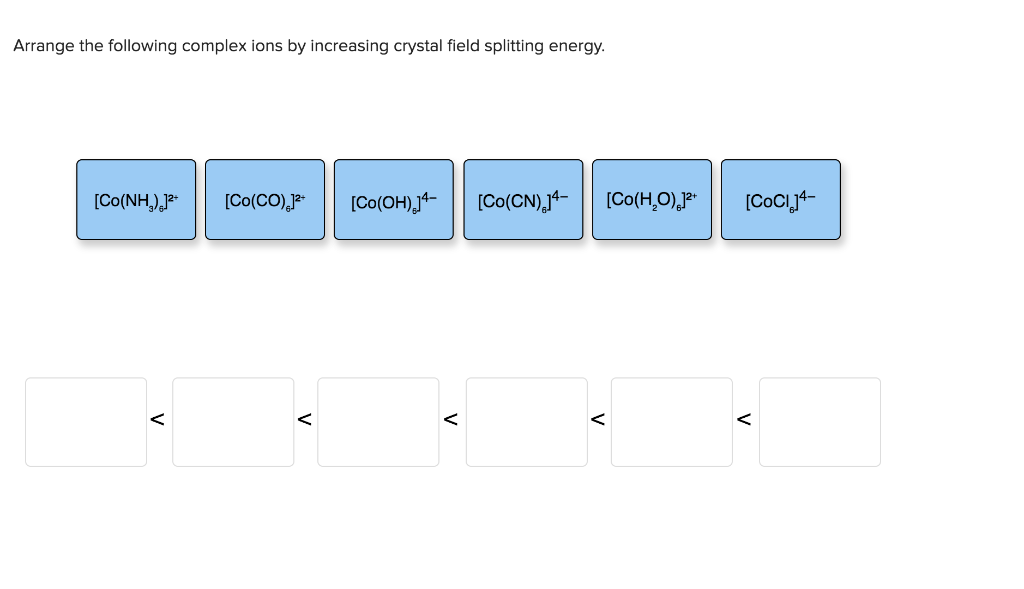Arrange the following complex ions by increasing crystal field splitting energy. [Co(NH) [Co(CO), [Co(OH)34- [Co(CN),J4- [COCH,O),P [COCIJA-